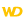Home |Sign in | FrenchHelpWLanguageWLanguage functionsControls, pages and windowsDrawing functionsPrefixed syntaxDrawing functions on WDPic variablesDrawArcPresentationExampleCoordinates of the arcDrawing a solid figureDrawing in PHPDrawing in Browser codeRelated examplesSee alsoOpenSaveAlphaBlendBackgroundChangeModeDrawArcDrawChordDrawCircleDrawLineDrawPointDrawPolygonDrawPolylineDrawRectangleDrawRectangleGradientDrawRoundedRectangleDrawSliceDrawTextDrawTextRTFEndDrawingFillFontHorizontalSymmetryInvertColorMergeLayerPixelColorPixelOpacityResizeRotationSaveStartDrawingVerticalSymmetryPicMergeLayerPicOpenPicResizePicRotationPicSaveNew WINDEV and WEBDEV 25 feature!
• Coordinates of the arc
• Drawing a solid figure
• Drawing in PHP
• Drawing in Browser code
WINDEVWEBDEVWINDEV MobileOthers<WDPic variable>.DrawArc (Function)
In french: <Variable WDPic>.DessineArc
Draws a circle arc on an ellipse arc in a WDPic variable.
The arc is drawn anticlockwise.Versions 15 and laterThis function is now available for WEBDEV sites in Linux.New in version 15This function is now available for WEBDEV sites in Linux.This function is now available for WEBDEV sites in Linux.
Example
MyWDPic is WDPic = "Test.gif"
// Draw a light yellow arc of circle in MyWDPic variable
MyWDPic.DrawArc(10, 10, 50, 50, 20, 20, 40, 50, LightYellow)

IMG_MyDrawing = MyImage
Syntax

Drawing an arc

<WDPic image>.DrawArc(<X1> , <Y1> , <X2> , <Y2> , <X3> , <Y3> , <X4> , <Y4> [, <Line color> [, <Line thickness>]])
<WDPic image>: WDPic variable
Name of the WDPic variable to use. Only the background layer will be handled.
<X1>: Integer
X coordinate (in pixels) of top left corner of the rectangle containing the circle.
<Y1>: Integer
Y coordinate (in pixels) of top left corner of the rectangle containing the circle.
<X2>: Integer
X coordinate (in pixels) of bottom right corner of the rectangle containing the circle.
<Y2>: Integer
Y coordinate (in pixels) of bottom right corner of the rectangle containing the circle.
<X3>: Integer
X coordinate (in pixels) of start point of the arc of circle.
<Y3>: Integer
Y coordinate (in pixels) of start point of the arc of circle.
<X4>: Integer
X coordinate (in pixels) of end point of the arc of circle.
<Y4>: Integer
Y coordinate (in pixels) of end point of the arc of circle.
<Line color>: Integer or constant (optional)
Color of the arc of circle. This color can correspond to:
• an RGB color (returned by RGB),
• an HSL color (returned by HSL),
• a preset color of WLanguage,
•a Color variable.
If this parameter is not specified, the line color:
<Line thickness>: Optional integerThickness (in pixels) of the arc of circle to draw. If this parameter is not specified, the value taken into account can be:
Remarks

Coordinates of the arc

If the start and end points are not located on the border of the circle (or on the border of the ellipse), the point taken into account corresponds to the intersection between the circle and the line that joins the specified point and the center of the rectangle (which means the center of the circle).The origin used to calculate the coordinates corresponds to the top left corner of the image (coordinates: (0,0)).

Drawing a solid figure

To draw a solid figure (a full arc), use <WDPic variable>.DrawChord or <WDPic variable>.DrawSlice.
Related Examples:Unit examples (WINDEV): The drawing functions [ + ] Using the main drawing functions of WINDEV to:- Initialize an Image control for drawing- Draw simple shapes- Write a text into a drawing- Change the color in a drawing
Business / UI classification : Neutral code
Component : wd250pnt.dll
Minimum version required
• Version 25